## Chapter 7Spin Relaxation

### 7.1 Strain Dependence of Surface Roughness Limited Spin Relaxation Matrix Elements

The surface roughness induced spin relaxation matrix elements are calculated between wave functions with opposite spin projections. The matrix elements are calculated in the same manner as in Chapter 6, i.e. the spin relaxation matrix elements normalized to the value of the intrasubband scattering at zero strain are written as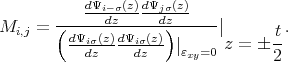(7.1)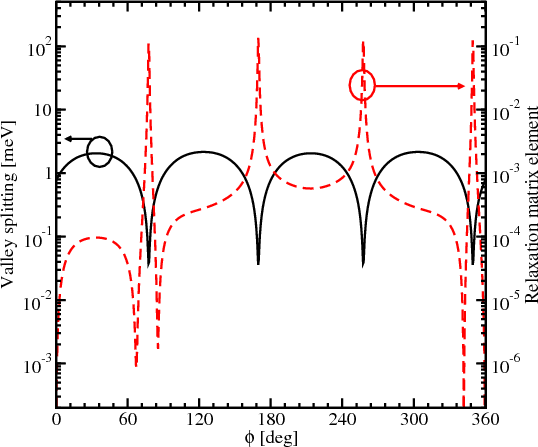Figure 7.1: Dependence of the normalized spin relaxation matrix elements and valley splitting on the angle between the incident and scattered waves for the quantum well thickness is 4nm, the conduction band offset is 4eV, kx=0.5nm-1, k y=0.1nm-1, E field=0MV/cm, ε=0.01%.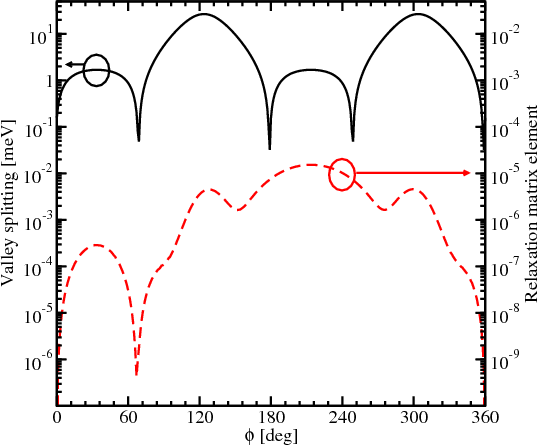Figure 7.2: Dependence of the normalized spin relaxation matrix elements and valley splitting on the angle between the incident and scattered waves for the quantum well thickness is 4nm, the conduction band offset is 4eV, kx=0.5nm-1, k y=0.1nm-1, E field=0MV/cm, ε=0.92%.

Figure 7.1 and Figure 7.2 show the spin relaxation matrix elements (normalized to intravalley scattering at zero strain) on the angle between the incident and scattered wave vectors together with the valley splitting for the scattered wave. Oscillations of the valley splitting are observed. In Figure 7.1 the sharp increase of the relaxation matrix element is correlated with the reduction of the valley splitting which occurs for the values of the angle determined by zeroes of the xy -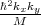term. This is the condition of the formation of the so called spin hot spots characterized by the maximum spin mixing. In contrast to Figure 7.1, the valley splitting reduction due to the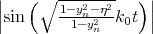term shown in Figure 7.2 does not lead to a sharp increase of the spin relaxation matrix elements on the angle between the incident and scattered waves.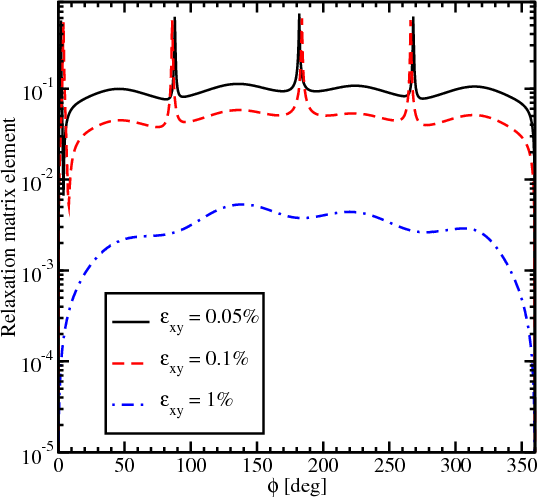Figure 7.3: Dependence of the normalized spin relaxation matrix elements on the angle between the incident and the relaxed wave for different values of strain for kx=0.8nm-1, k y=0, t=4nm, ΔEC=10eV.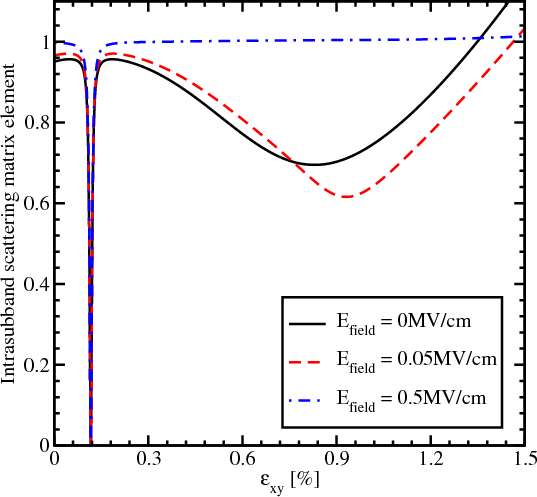Figure 7.4: Intravalley scattering matrix elements normalized by their values for zero strain as a function of shear strain for different electric field values.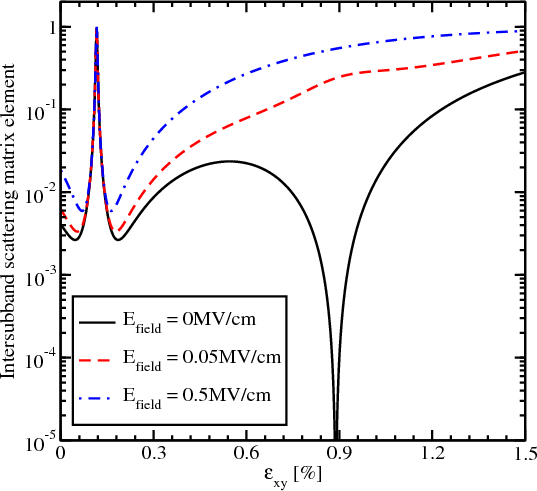Figure 7.5: Intervalley scattering matrix elements normalized to the value of the intravalley scattering at zero strain as a function of strain for different electric field values.

The splitting between the subbands depends on xy - kxky∕M and their degeneracy is lifted by the kinetic-like term kxky∕M even without shear strain. This results in a strong dependence of the surface roughness induced spin relaxation matrix elements on the angle between the incident and outgoing wave vectors (Figure 7.1 and Figure 7.2). Figure 7.3 shows the dependences of the relaxation matrix elements on this angle for different values of shear strain. The sharp peaks in Figure 7.3 for shear strain values 0.05% and 0.1% nature of which were discussed for Figure 7.1 and Figure 7.2 are observed for similar angle values. However, the intersubband matrix relaxation elements for higher strain show lower values, except peaks. For the shear strain of 1% no peaks are observed and the matrix element values are smaller by several orders of magnitude. As follows from Figure 7.3, shear strain leads to the reduction of the spin relaxation matrix element.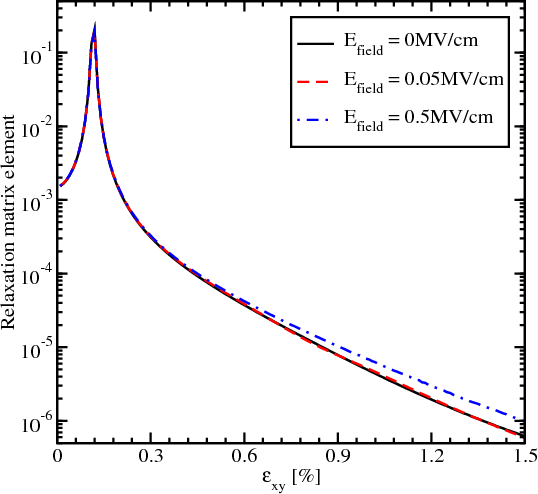Figure 7.6: Spin relaxation matrix elements normalized to intravalley scattering at zero strain dependence on shear strain for several values of the electric field, kx=0.5nm-1, k x=0.1nm-1.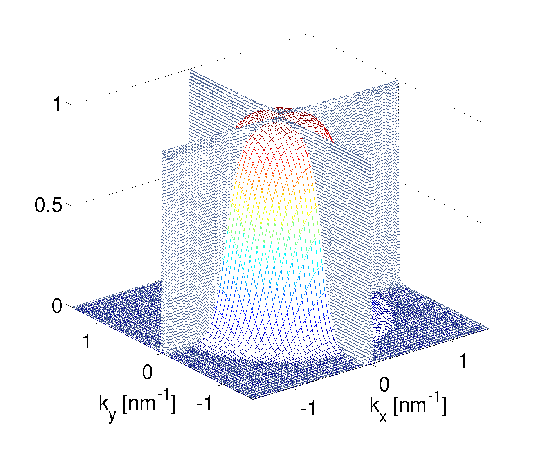Figure 7.7: Intersubband relaxation matrix elements normalized to the intrasubband scattering elements at zero strain for an unstrained sample. The Fermi distribution for 300K is also shown.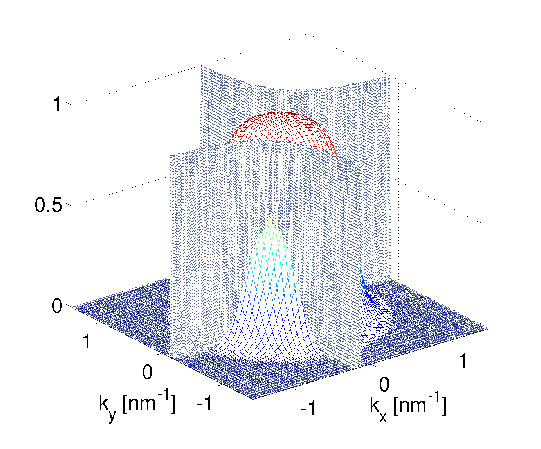Figure 7.8: Normalized intersubband relaxation matrix elements for shear strain 0.5% shown together with the Fermi distribution at 300K.

Figure 7.4 and Figure 7.5 show the dependences on strain and electric field of the matrix elements for the intrasubband and intersubband scattering. The intrasubband scattering matrix elements have two decreasing regions shown in Figure 7.4. These regions are in good agreement with the valley splitting minima in Figure 5.19. For higher fields the second decreasing region around the shear strain value of 0.931% vanishes. For the electric field of 0.5MV/cm the intrasubband matrix elements are sharply reduced only for the shear strain value of 0.116%. At the same time, the intersubband matrix elements show a sharp increase around the shear strain value of 0.116%. The electric field does not affect much the valley splitting provided by the zero value of the term xy -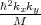, and the sharp increase in the intersubband matrix elements is observed at higher fields. At the same time the electric field washes out a sharp minimum around the shear strain value of 0.931% in Figure 7.5. With the electric field increased the confinement pushes the carriers closer to the interface which results in both an intersubband and an intrasubband scattering matrix elements increase.

The dependence of the spin relaxation matrix elements on shear strain for several values of the electric field is shown in Figure 7.6. The spin relaxation matrix elements increase until the strain value 0.116%, the point determined by the spin hot spot condition. Applying strain larger than 0.116% suppresses the spin relaxation significantly, for all values of the electric field. In contrary to the scattering matrix elements (Figure 7.4 and Figure 7.5), the relaxation matrix elements demonstrate a sharp feature only for the shear strain value of 0.116% at zero electric field. Large electric field leads to an increase of the relaxation matrix elements due to the additional field-induced confinement resulting in higher values of the surface roughness induced spin relaxation matrix elements.

Figure 7.7 displays the normalized spin relaxation matrix elements for an unstrained film. The "hot spots" are along the  and  directions. Shear strain pushes the "hot spots" to higher energies (Figure 7.8) outside of the states occupied by carriers. This leads to a reduction of the spin relaxation.

### 7.2 Spin Relaxation Rate

#### 7.2.1 Surface Roughness Induced Spin Relaxation Rate

The surface roughness spin relaxation rate is calculated in the following way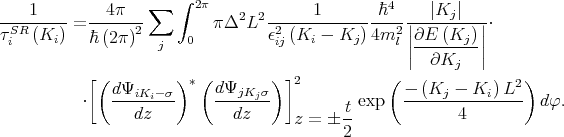(7.2)

Ki,Kj are the in-plane wave vectors before and after scattering, φ is the angle between Ki and Kj, ϵ is the dielectric permittivity, L is the autocorrelation length, Δ is the mean square value of the surface roughness fluctuations, ΨiKi and ΨjKj are the wave functions, and σ = ±1 is the spin projection to the  axis.

#### 7.2.2 Phonons

All atoms in an ideal crystal lattice are fixed at their periodic positions. However, ions in non-ideal lattices oscillate around their fixed position. The oscillations depend on the lattice temperature and are called ion temperature oscillations. The nature of such oscillations is described by phonons. Since phonons have an oscillating nature a characteristic frequency ωq can be associated with them. The energy of a phonon is characterized by the frequency ωq is ωq. Oscillation are usually considered for a primitive cell. If the center mass of the cell during oscillations does not move then consider optic phonons, otherwise - acoustic phonons . The number of optic modes for the primitive cell that contains p atoms is determined by the expression 3(p - 1), while the number of acoustic modes is always 3. Each of the modes has three components - two transversal (TA1, TA2, TO1, TO2) and one longitudinal (LA, LO).

Following Ehrenreich and Overhauser  the phonons polarization vectors are written as(7.3)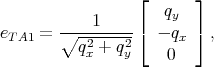(7.4)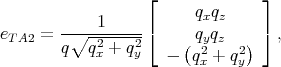(7.5)

with q =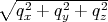.

Spin relaxation rates for transitions from band i to band j by mechanism m (i.e., acoustic phonon or optical phonon), are calculated using Fermi’s second Golden Rule(7.6)

where M is the momentum scattering (spin relaxation) matrix element, the material volume is chosen as the unit volume, 4π for spin relaxation accounts for the fact that the net number of spin polarized electrons changes by two with each spin flip .

#### 7.2.3 Intravalley and g-Intervalley Relaxation Processes Rates

The acoustic phonons scattering rate for the wave vector Ki in subband i is written as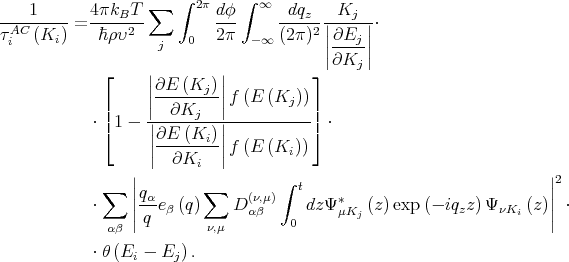(7.7)

Here, q =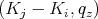is the phonon wave vector, Dαβ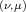is the deformation potential, α,β = {x,y}, eβ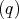= {eLA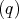,eTA1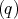,eTA2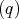} is the polarization vector.

By applying Fubini’s theorem the modulus in Equation 7.7 is replaced with the repeated integral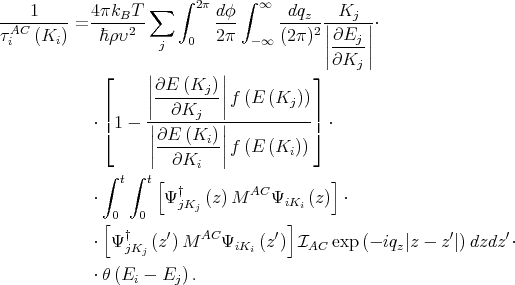(7.8)

where MAC is the deformation potential matrix, the exact form of the matrix depends on phonon mode and is given further, the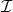AC term depends on the spin-flip process and the phonon mode . By replacing the order of the integration in Equation 7.8 the acoustic phonon relaxation rate is written as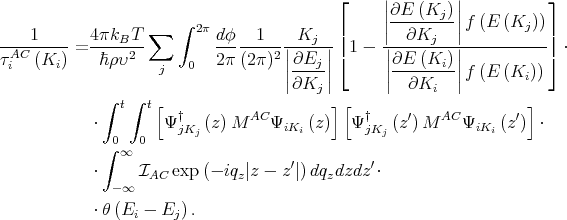(7.9)

Intervalley g-Process Spin Relaxation. The g-process describes scattering between opposite valleys. Following  theAC 1 for intervalley scattering. By taking into account the Dirac delta function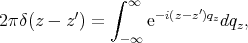(7.10)

and applying the following property of the Dirac delta function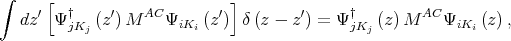(7.11)

Equation 7.9 is simplified to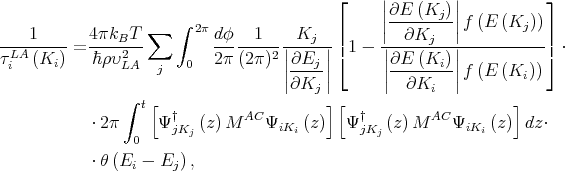(7.12)

where υLA = 8700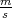, the matrix MAC (for the intervalley g-process spin relaxation denote it by M) contains the Elliott and Yafet contributions  is written as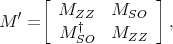(7.13)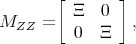(7.14)(7.15)

where Ξ = 12eV is the acoustic deformation potential,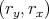= Ki + Kj, and DSO = 15meV/k0  with k0 = 0.15 2π∕a which is defined as the position of the valley minimum relative to the X-point in unstrained silicon.

Intravalley Transversal Acoustic Phonons Spin Relaxation. Intrasubband transitions are important for the contributions determined by the shear deformation potential. TheAC due to the transversal acoustic phonons is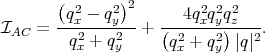(7.16)

Applying the theory of residues with Q2 = q x2 + q y2, the following integral is calculated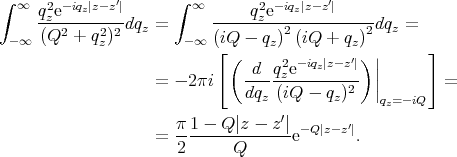(7.17)

The matrix MAC for the intrasubband transversal acoustic phonons (M) is written as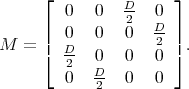(7.18)

Here D=14eV is the shear deformation potential.

Then Equation 7.9 for the intrasubband transversal acoustic phonons is then written as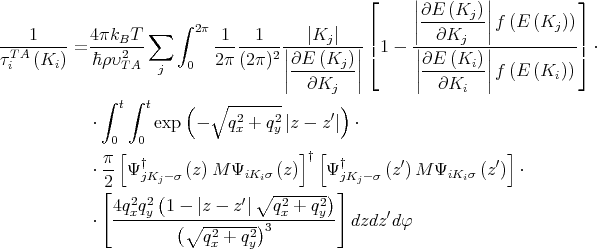(7.19)

where ρ=2329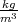is the silicon density , υTA=5300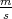is the transversal phonons’ velocity,= Ki - Kj.

Intravalley Longitudinal Acoustic Phonon Spin Relaxation. TheAC due to the longitudinal acoustic phonon is given by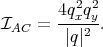(7.20)

Applying theory of residues with Q2 = q x2 + q y2, the following integral is calculated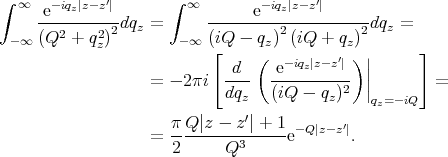(7.21)

The matrix MAC for the intrasubband longitudinal acoustic phonons is the same as in Equation 7.18.

From this follows, the intravalley spin relaxation rate due to longitudinal acoustic phonons is calculated as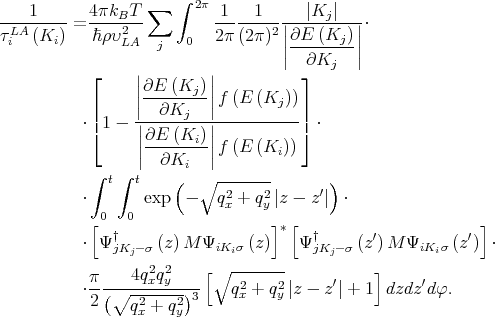(7.22)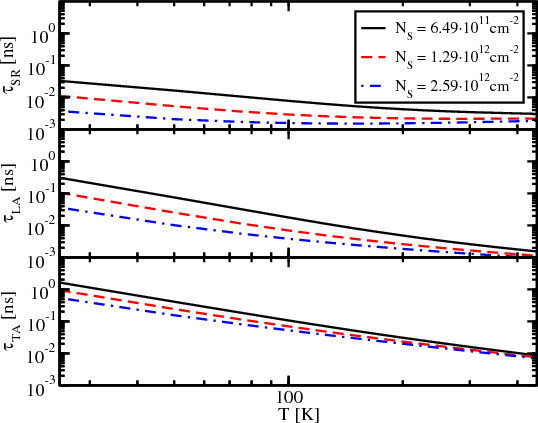Figure 7.9: Dependence of the surface roughness (SR), the longitudinal phonons’ (LA), and the transversal phonons’ (TA) contribution to the spin lifetime on temperature for different values of the electron concentration, for εxy=0, and film thickness 2.1nm.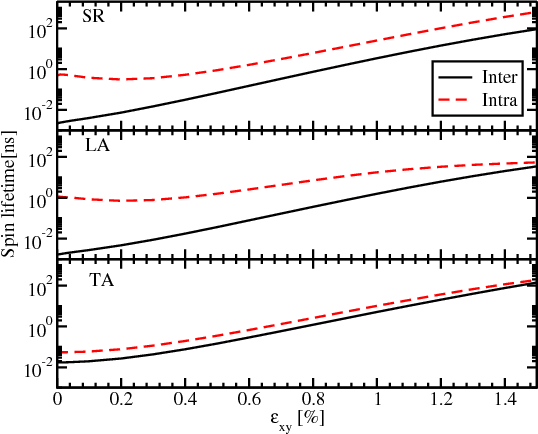Figure 7.10: Dependence of the intersubband and intrasubband components of the momentum relaxation time induced by surface roughness (SR) and acoustic phonons (PH) on shear strain for the film thickness 1.36nm, T=300K, and electron concentration 1.291012cm-2.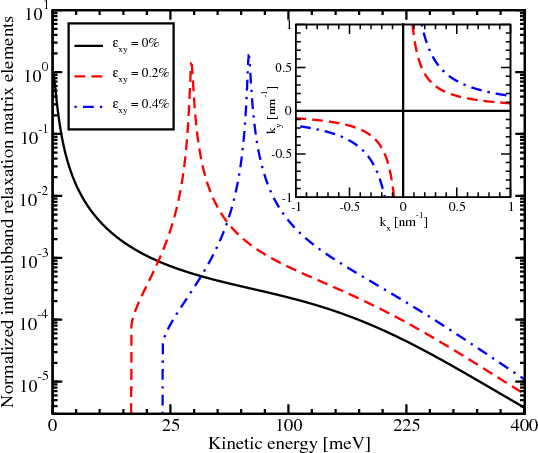Figure 7.11: Normalized intersubband relaxation matrix elements as a function of the conduction electrons kinetic energy in  direction. The inset shows the positions of the hot spots for different values of shear strain.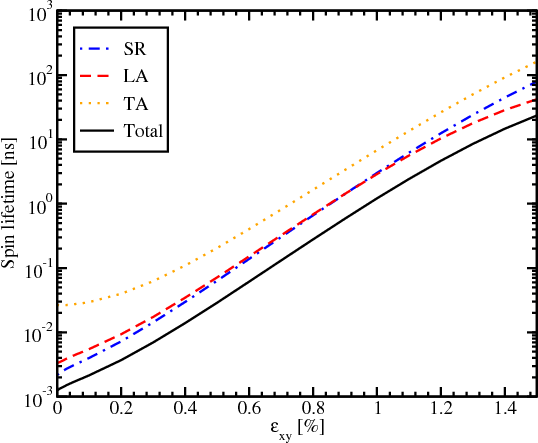Figure 7.12: Dependence of the spin lifetime on shear strain for the film thickness 2.1nm, T=300K, and electron concentration 1.291012cm-2.

The dependence of the spin lifetime on temperature for phonon scattering, and surface roughness scattering for different carrier concentrations is shown in Figure 7.9. The spin relaxation is more efficient for higher carrier concentrations for all three considered mechanisms. While the temperature increases, the difference between the spin lifetimes for different values of the electron concentration becomes less pronounced. Figure 7.9 also shows that the surface roughness mechanism dominates for all concentration values. Spin relaxation due to transversal acoustic phonons is the weakest among the three considered mechanisms.

Figure 7.10 demonstrates that the main contribution to spin relaxation comes from the intersubband processes due to the presence of the spin hot spots characterized by the sharp peaks of the intersubband spin relaxation matrix elements. Their position is shown in Figure 7.11. For higher shear strain values the hot spots are pushed to higher energies, away from the subband minima (inset in Figure 7.11). This results in a strong increase of the spin lifetime with shear strain for surface roughness and the phonon mechanisms as shown in Figure 7.12.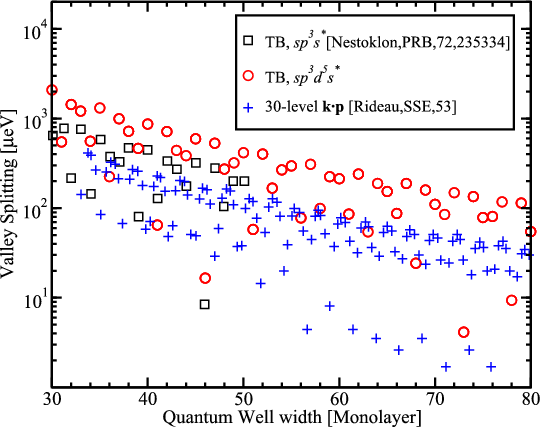Figure 7.13: Valley splitting in a Si quantum well at zero strain as a function of the quantum well width including results from literature .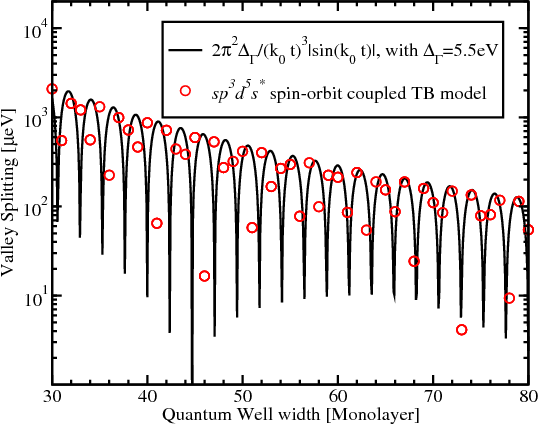Figure 7.14: Dependence of the valley splitting on the quantum well width from the tight binding (TB) model and the analytical expression with ΛΓ=5.5eV.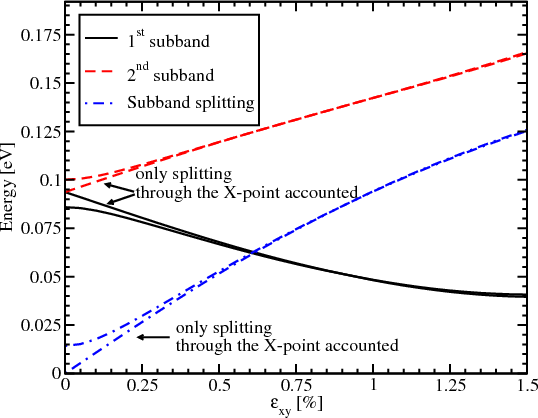Figure 7.15: Dependence of energy of the 1st and the 2nd subband together with the subband splitting on shear strain for the film thickness of 2.1nm.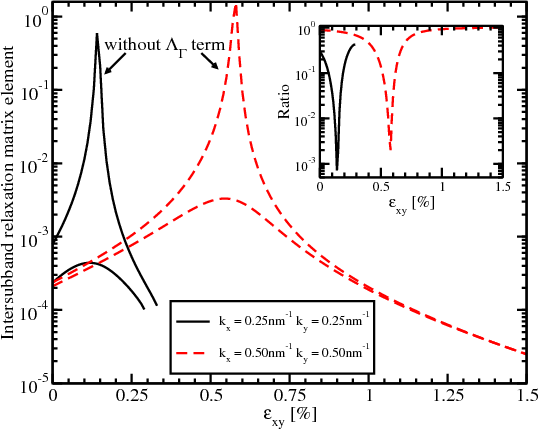Figure 7.16: Dependence of the normalized intersubband relaxation matrix elements on shear strain for 2.1nm film thickness. The inset shows the ratio of the matrix elements with the ΛΓ term to the matrix elements without the ΛΓ term.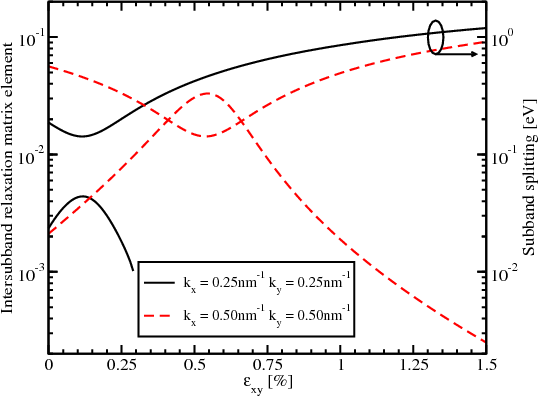Figure 7.17: Normalized intersubband relaxation matrix elements and subband splitting as a function of shear strain calculated with taking into account zero-strain splitting.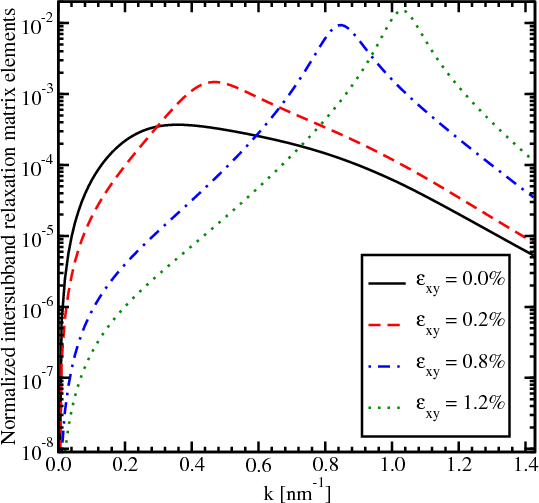Figure 7.18: Normalized intersubband spin relaxation matrix elements as a function of the wave vector in  direction.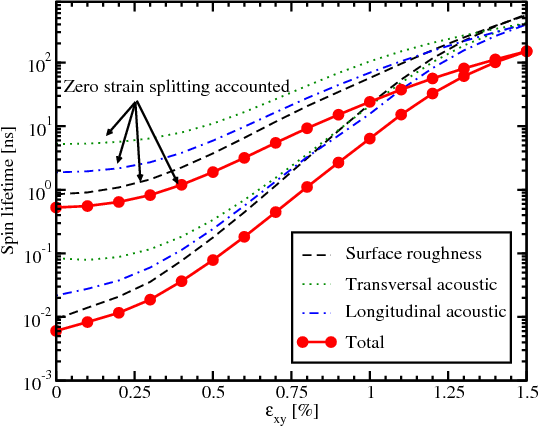Figure 7.19: Dependence of spin lifetime on shear strain for T=300K and a film thickness of 2.1nm.

### 7.4 Valley Splitting in Unstrained Films

The  equivalent valley coupling through the Γ-point results in a subband splitting in confined electron structures . The values of the valley splitting obtained from a 30-band kp model , an atomistic tight-binding model , and sp3d5s* code are shown in Figure 7.13. Although looking irregular, the results follow a certain law. Figure 7.14 demonstrates a good agreement of the results of the tight-binding calculations with the analytical expression for the subband splitting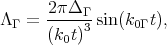(7.23)

where ΔΓ is the splitting at Γ point,k = 0.85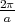, a is the lattice constant, and t is the film thickness. The good agreement is found for the value ΔΓ=5.5eV.

Figure 7.15 shows the dependence of the lowest unprimed subbands energies and their splitting on shear strain with and without accounting for the ΛΓ term. The unprimed subbands are degenerate at zero strain without the ΛΓ term. The ΛΓ term lifts the degeneracy while shear strain gives the major contribution to the splitting at high strain values. The surface roughness scattering matrix elements are taken to be proportional to the square of the product of the subband wave function derivatives at the interface . The electron-phonon scattering is accounted for by the deformation potential approximation . The surface roughness intersubband spin relaxation matrix elements with and without the ΔΓ term are shown in Figure 7.16. The difference in the matrix elements’ values calculated with and without the ΔΓ term (inset Figure 7.16) can reach two orders of magnitude. Hence, the valley coupling through the Γ-point must be taken into account for the accurate spin lifetime calculations.

The hot-spot peaks shown in Figure 7.11 becomes smoother if the Γ-point splitting is considered (Figure 7.18). However, the peaks are still well-pronounced and follow the same trend.

The peaks on the matrix elements’ dependences (Figure 7.16) are correlated with the unprimed subband splitting minima (Figure 7.17). For higher strain values the peaks corresponding to strong spin relaxation hot spots are pushed towards unoccupied states at higher energies (Figure 7.17). This leads to the strong increase of the spin lifetime demonstrated in Figure 7.19. The increase is less pronounced, if the ΛΓ term responsible for the valley splitting in relaxed films is taken into account. However, even in this case the spin lifetime is boosted by almost two orders of magnitude.

### 7.5 Primed Subbands and f-Processes

#### 7.5.1 Primed k⋅p Hamiltonian

The primed subbands are governed by the following Hamiltonian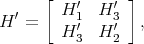(7.24)

where H1, H 2, and H 3 are written as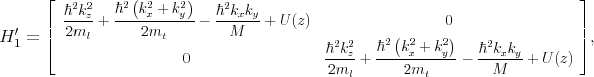(7.25)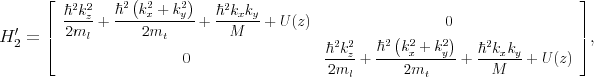(7.26)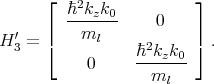(7.27)

A quantization along X-axis is assumed.

#### 7.5.2 f-Process Relaxation Rate

The f-process describes scattering between valleys that reside on perpendicular axes. The spin relaxation is calculated by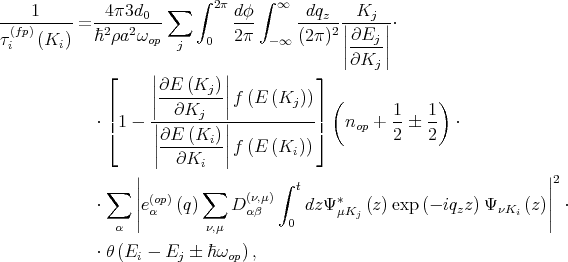(7.28)

a defines the silicon lattice constant, d0 is the optical deformation potential, ωop denotes the frequency of the optical phonons, and nop describes the Bose occupation factor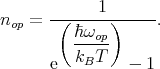(7.29)

The relaxation rate for the transition between primed and unprimed subbands is given by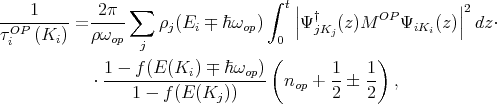(7.30)

ρj(E) is the density of states for subband j and MOP is written as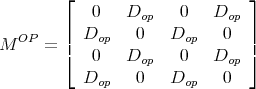(7.31)

with Dop = 6.5meV.

### 7.6 Results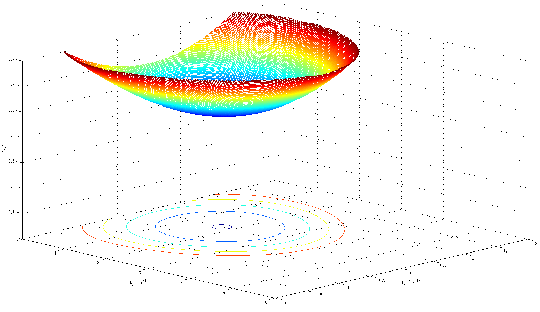Figure 7.20: Energy dispersion for the primed subband for 2.1nm film thickness.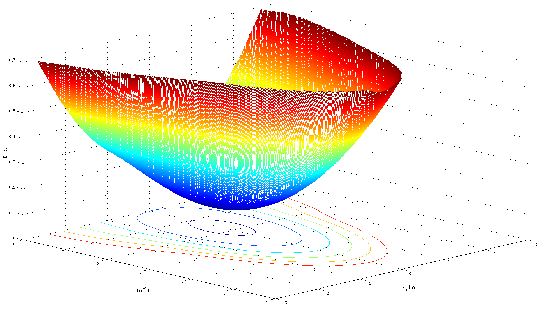Figure 7.21: Energy dispersion for the primed subband for 5nm film thickness.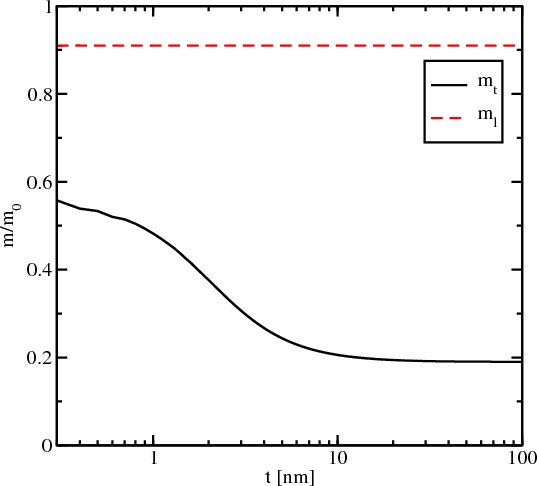Figure 7.22: Dependence of the effective mass for the lowest ground subband on thickness.

In Figure 7.20 and Figure 7.21 the dispersion of the first primed subband in films with thicknesses of 2.1nm and 5nm, respectively, is compared. The minimum energy of the subband is located at the point kz = k0, ky = 0, and its value for an infinite potential square well is determined by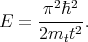(7.32)

The values of the subband minima for the well thickness of 2.1nm and 5nm are 0.44eV and 0.08eV, respectively. These values are in good agreement with Figure 7.20 and Figure 7.21.

The dependence of the longitudinal and transversal effective masses on the well thickness is shown in Figure 7.22. The longitudinal effective mass ml = 0.91m0 does not depend on the thickness. In sharp contrast, the effective mass mt shows a strong increase as the film thickness reduces, in agreement with earlier predictions .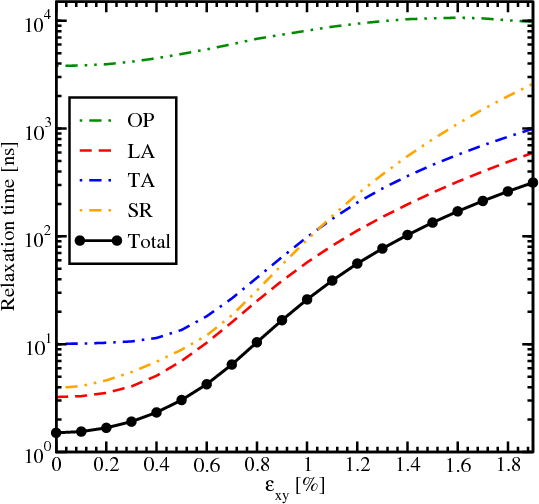Figure 7.23: Spin lifetime as a function of strain for 3nm film thickness and an electron concentration of 51012cm-2. Contributions due to optical, acoustic and surface roughness are included.Figure 7.24: Spin lifetime as a function of strain for 4nm film thickness and an electron concentration of 51012cm-2. Contributions due to optical, acoustic and surface roughness are included.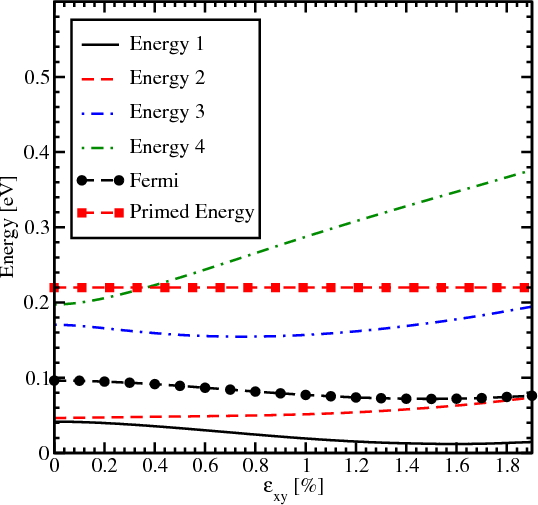Figure 7.25: Dependence of energies for primed, unprimed subbands, and Fermi energy on strain for 3nm film thickness.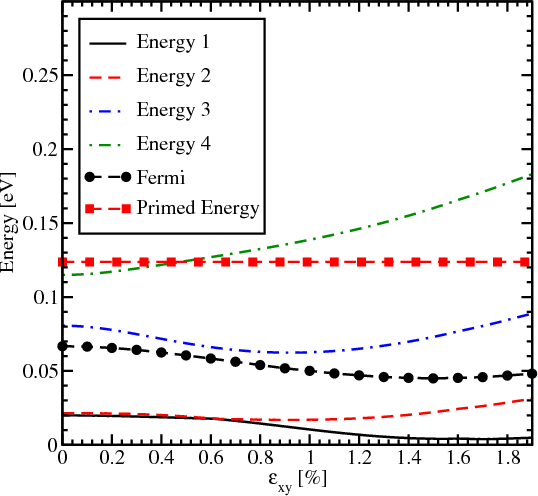Figure 7.26: Dependence of energies for primed, unprimed subbands, and Fermi energy on strain for 4nm film thickness.

Figure 7.23 and Figure 7.24 show the total spin lifetime and contributions due to acoustic, optical phonons, and surface roughness as a function of strain for two different thicknesses (3nm and 4nm). For the film of 3nm thickness the contribution due to longitudinal acoustic phonons (LA) is close to the contribution from surface roughness for small shear strain values. Further increase of strain makes LA phonons the main contributors to the total spin lifetime. For the thicker film shown in Figure 7.24 the total spin lifetime mainly follows the same trend as the longitudinal acoustic phonons spin relaxation. Interestingly, the intersubband optical phonons calculated as g-process can be safely ignored for the film of 3nm thickness, while their contribution for the film of 4nm thickness is more considerable. The dependence of g-process phonons on strain is not so significant as the surface roughness, intrasubband scattering, and f-process scattering. Thus, for thicker films the effect of several orders of magnitude increase of spin lifetime in strained films should not be observed.

The dependence of energies for primed and unprimed subbands on strain for kx = 0 and ky = 0 together with the Fermi energy is shown in Figure 7.25 and Figure 7.26 for 3nm and 4nm, respectively. Energy 1 and Energy 2 stand for the lowest subbands of the two opposite valleys along  direction. Energy 3 and Energy 4 stand for the second unprimed subbands. The increasing importance of the g-process shown in Figure 7.24 compared to  Figure 7.23 comes from the fact that the distance between the Fermi energy and the lowest energy in the primed subband decreases with thickness increasing. The occupation of the second subband become less pronounced at high strain as is confirmed by the Fermi level dependence for both thickness values (Figure 7.25 and Figure 7.26).